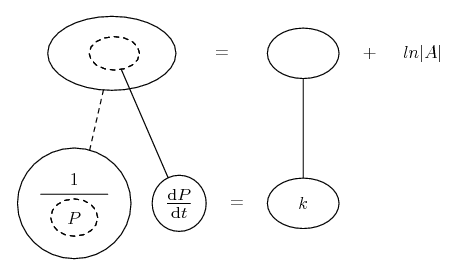# Thread: Bacteria Growth Problem

1. ## Bacteria Growth Problem

A bacteria culture initially contains P(o) cells and grows at the rate dP/dt = kP where k is a growth constant. After an hour the population has doubled.

(a) Determine an expression for the number of bacteria present after t hours.

(b) Computer the number of bacteria present, and the rate of growth, after 2 hours.

(c) After how many hours will the population be 10P(o)

I have no idea how to do this, thanks for any help!

2. Do you know how to 'separate variables'? In this case, divide both sides by P and integrate both sides with respect to t, using the chain rule on the left

3.Originally Posted by SakasA bacteria culture initially contains P(o) cells and grows at the rate dP/dt = kP where k is a growth constant. After an hour the population has doubled.

(a) Determine an expression for the number of bacteria present after t hours.
You can "separate" the differential equation dP/dt= kP as dp/P= kdt. Integrate both sides of that. You will, of course, have a "constant of integration". You can use the fact that "After an hour the population has doubled", P(1)= 2P(0), to find k.

(b) Computer the number of bacteria present, and the rate of growth, after 2 hours.
Once you have done that, just set t= 2 and do the arithmetic.

(c) After how many hours will the population be 10P(o)
Set P(t)= 10P(0) and solve for t.

I have no idea how to do this, thanks for any help!

4.Originally Posted by HallsofIvyYou can "separate" the differential equation dP/dt= kP as dp/P= kdt. Integrate both sides of that. You will, of course, have a "constant of integration". You can use the fact that "After an hour the population has doubled", P(1)= 2P(0), to find k.

Once you have done that, just set t= 2 and do the arithmetic.

Set P(t)= 10P(0) and solve for t.
How would I do (a) without integration, since we haven't learned that yet?

5. Er, I suppose that could conceivably be the case if you're being familiarised first with equations of the form P = Ae^(kt), which is what you'll get in this case, and from which you can follow HoI's advice.

Anyhow... have you learnt differentiation? Now you're reversing the process. You 'separate variables' so you can integrate (anti-differentiate) both sides of your equation with respect to t, but using the chain rule on the left hand side. Just in case a picture helps...... where... is the chain rule. Straight continuous lines differentiate downwards (integrate up) with respect to x, and the straight dashed line similarly but with respect to the dashed balloon expression (the inner function of the composite, which is subject to the chain rule).

Can you remember what function of P i.e. ?(P) it is whose derivative (with respect to P) is 1/P ? And what's the function ?(t) whose derivative (differentiating with respect to t) is a constant (i.e. k) ?

The plus ln|A| there on the right is called the 'constant of integration'. Calling it 'ln|A|' instead of the usual 'A' will possibly help (a bit) when you try to simplify the top row...

__________________________________________

Don't integrate - balloontegrate!

Balloon Calculus: Gallery

Balloon Calculus Drawing with LaTeX and Asymptote!

,

,

,

### growth problem about bacteria

Click on a term to search for related topics.

#### Search Tags

bacteria, growth, problem